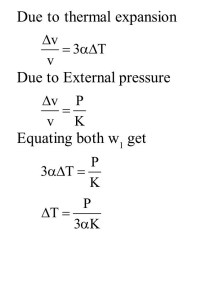# An external pressure

Question:

An external pressure $P$ is applied on a cube at $0^{\circ} \mathrm{C}$ so that it is equally compressed from all sides. $K$ is the bulk modulus of the material of the cube and $\alpha$ is its coefficient of linear expansion. Suppose we want to bring the cube to its original size by heating. The temperature should be raised by :

1. $\frac{3 \alpha}{P K}$

2. $3 P K \alpha$

3. $\frac{\mathrm{P}}{3 \alpha K}$

4. $\frac{\mathrm{P}}{\alpha \mathrm{K}}$

Correct Option: , 3

Solution: